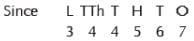# Olympiad Test: Number System -2

Test Description

## 10 Questions MCQ Test Mathematics Olympiad Class 4 | Olympiad Test: Number System -2

Olympiad Test: Number System -2 for Class 4 2023 is part of Mathematics Olympiad Class 4 preparation. The Olympiad Test: Number System -2 questions and answers have been prepared according to the Class 4 exam syllabus.The Olympiad Test: Number System -2 MCQs are made for Class 4 2023 Exam. Find important definitions, questions, notes, meanings, examples, exercises, MCQs and online tests for Olympiad Test: Number System -2 below.
 1 Crore+ students have signed up on EduRev. Have you?
Olympiad Test: Number System -2 - Question 1

### Instead of putting comma, we can _____________ to separate the periods.

Detailed Solution for Olympiad Test: Number System -2 - Question 1

Instead of putting commas, we can leave space to separate the periods.

Olympiad Test: Number System -2 - Question 2

### Ten lakhs comes in _________ period.

Detailed Solution for Olympiad Test: Number System -2 - Question 2

Ten lakh comes in lakhs period.

Olympiad Test: Number System -2 - Question 3

### Pick odd one out:

Detailed Solution for Olympiad Test: Number System -2 - Question 3

12,34,56 is an even number. The rest all are odd numbers.

Olympiad Test: Number System -2 - Question 4

Pick the different one out:

Detailed Solution for Olympiad Test: Number System -2 - Question 4

The answer is D because there is a different way of putting commas.

Olympiad Test: Number System -2 - Question 5

Pick the odd one out:

Detailed Solution for Olympiad Test: Number System -2 - Question 5

Because it has a different way of putting commas.

Olympiad Test: Number System -2 - Question 6

3,44,567 has ______________ lakhs.

Detailed Solution for Olympiad Test: Number System -2 - Question 6

3,44,567 has 3 lakhs.Olympiad Test: Number System -2 - Question 7

3,44,567 has _____________ thousands

Detailed Solution for Olympiad Test: Number System -2 - Question 7the number has 4 on thousand's place

Olympiad Test: Number System -2 - Question 8

3,44,567 has ______________ ones

Detailed Solution for Olympiad Test: Number System -2 - Question 8so it 7 has ones.

Olympiad Test: Number System -2 - Question 9

3,44,567 has ______________ tens

Detailed Solution for Olympiad Test: Number System -2 - Question 9so it has 6 tens

Olympiad Test: Number System -2 - Question 10

3,44,567 has _____________ hundreds

Detailed Solution for Olympiad Test: Number System -2 - Question 10

The correct answer is C = 3,44,567

2nd is tens ,3rd is hundreds , 4th is th.5th is tth. 6 th is lakh. So we can conclude that 5 is in hundred's places.